# 【讨论】Graphics 画线纹理的一种简单实现！(新增绳子纹理效果)

【本文参与征文活动】

`cc.Graphics` 画线也能加纹理了？文末附送完整代码。

`Graphics` 中有一个 `_impl` 变量。

`lineTo``moveTo` 都会往 `_paths` 塞入画线的点数据。

``````for (let index = 0; index < _impl._paths.length; index++) {
const path = _impl._paths[index];
const pathPoints = path.points;
if (pathPoints.length < 2) continue;
for (let index2 = 1; index2 < pathPoints.length; index2++) {
// 当前点
const p = cc.v2(pathPoints[index2].x, pathPoints[index2].y);
// 上一个点
const p_pre = cc.v2(pathPoints[index2 - 1].x, pathPoints[index2 - 1].y);
}
}
``````

``````const dir = p.sub(p_pre); //方向
``````

``````const cross_dir = (dir.y == 0 ? cc.v2(0, 1) : cc.v2(1, -dir.x / dir.y).normalize()).mulSelf(w / 2); //垂直方向
``````

``````const p_r_t = p.add(cross_dir); //右上
const p_r_b = p.sub(cross_dir); // 右下
const p_l_t = p_pre.add(cross_dir); // 左上
const p_l_b = p_pre.sub(cross_dir); // 左下
``````

``````const uv_mul = 50;
const i_offset = vertices.x.length;
vertices.x.push(p_r_t.x, p_r_b.x, p_l_t.x, p_l_b.x);
vertices.y.push(p_r_t.y, p_r_b.y, p_l_t.y, p_l_b.y);
vertices.nu.push(p_r_t.x / uv_mul, p_r_b.x / uv_mul, p_l_t.x / uv_mul, p_l_b.x / uv_mul);
vertices.nv.push(p_r_t.y / uv_mul, p_r_b.y / uv_mul, p_l_t.y / uv_mul, p_l_b.y / uv_mul);

vertices.triangles.push(i_offset + 0);
vertices.triangles.push(i_offset + 1);
vertices.triangles.push(i_offset + 2);
vertices.triangles.push(i_offset + 1);
vertices.triangles.push(i_offset + 2);
vertices.triangles.push(i_offset + 3);
``````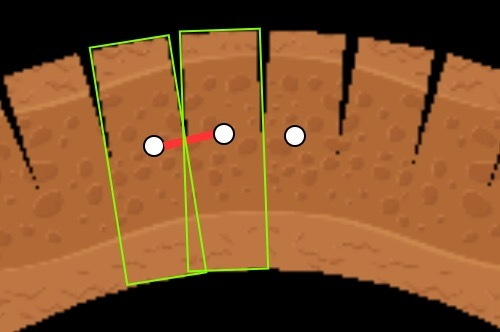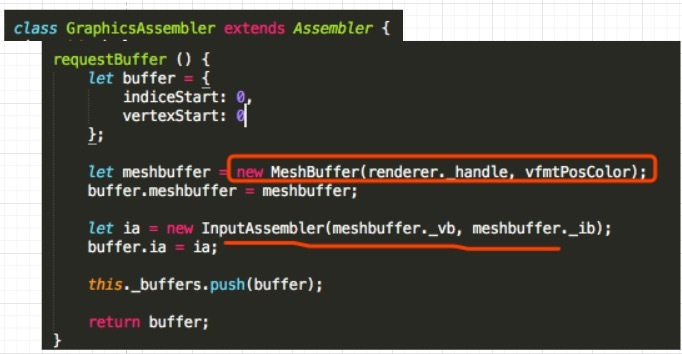# 画线纹理之连接优化``````// 角度
const r = 2 * Math.PI * index3 / count;
// 先算方向向量，在加上圆心坐标就是，圆上的点。
const pos_circle = cc.v2(w / 2 * Math.cos(r), w / 2 * Math.sin(r)).addSelf(p);
``````

``````//画圆
const count = 12;
i_offset = vertices.x.length;
vertices.x.push(p.x);
vertices.y.push(p.y);
vertices.nu.push(p.x / uv_mul);
vertices.nv.push(p.y / uv_mul);
for (let index3 = 0; index3 < count; index3++) {
const r = 2 * Math.PI * index3 / count;
const pos_circle = cc.v2(w / 2 * Math.cos(r), w / 2 * Math.sin(r)).addSelf(p);
vertices.x.push(pos_circle.x);
vertices.y.push(pos_circle.y);
vertices.nu.push(pos_circle.x / uv_mul);
vertices.nv.push(pos_circle.y / uv_mul);
if (index3 === 0) {
// 0 - count -1
vertices.triangles.push(i_offset, i_offset + 1 + index3, i_offset + count);
} else {
// 0 - index3 - (index3-1)
vertices.triangles.push(i_offset, i_offset + 1 + index3, i_offset + index3);
}
}
``````# 画线纹理之绳子

## 效果预览## 前置教程## 实现原理``````// 从最后一点开始计算
for (let index2 = pathPoints.length - 1; index2 > 0; index2--) {
// 省略部分代码
vertices.x.push(p_r_t.x, p_r_b.x, p_l_t.x, p_l_b.x);
vertices.y.push(p_r_t.y, p_r_b.y, p_l_t.y, p_l_b.y);
// 计算uv
vertices.nu.push(offsetX.x * uv_mul, offsetX.x * uv_mul, (offsetX.x + dirLen) * uv_mul, (offsetX.x + dirLen) * uv_mul);
vertices.nv.push(1, 0, 1, 0);
// 省略部分代码
offsetX.addSelf(cc.v2(dirLen, 0)); // 记录已经画的长度长度
}
````````````let trianglesCache: number[][] = [];
for (let index2 = pathPoints.length - 1; index2 > 0; index2--) {
// 省略部分代码
triangles.push(i_offset + 0);
triangles.push(i_offset + 1);
triangles.push(i_offset + 2);
triangles.push(i_offset + 1);
triangles.push(i_offset + 2);
triangles.push(i_offset + 3);
trianglesCache.push(triangles);
}
trianglesCache.reverse(); // 顶点索引反转
trianglesCache.forEach(v => {
// 真正的顶点索引顺序
vertices.triangles.push(...v)
})
````````````//画圆
const dir_angle = dir.signAngle(cc.v2(-1, 0));//与x轴的负方向的夹角
const count = 12;
i_offset = vertices.x.length;
// 这里是圆心
vertices.x.push(p.x);
vertices.y.push(p.y);
vertices.nu.push(offsetX.x * uv_mul);
vertices.nv.push(0.5);
for (let index3 = 0; index3 < count; index3++) {
const r = 2 * Math.PI * index3 / count;
// 圆心到各个边的向量
const pos_circle = cc.v2(w / 2 * Math.cos(r), w / 2 * Math.sin(r));
// 对于圆的uv需要旋转
vertices.nu.push((pos_circle.rotate(dir_angle).x + offsetX.x) * uv_mul);
vertices.nv.push(pos_circle.rotate(dir_angle).y / w + 0.5);
if (index3 === 0) {
triangles.push(i_offset, i_offset + 1 + index3, i_offset + count);
} else {
triangles.push(i_offset, i_offset + 1 + index3, i_offset + index3);
}
}
``````## 小结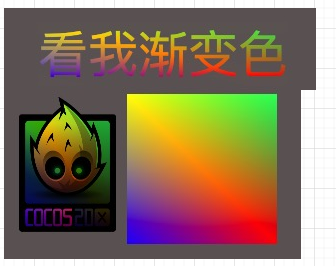36赞

mark…强！学到了最终决定在交接处画一个圆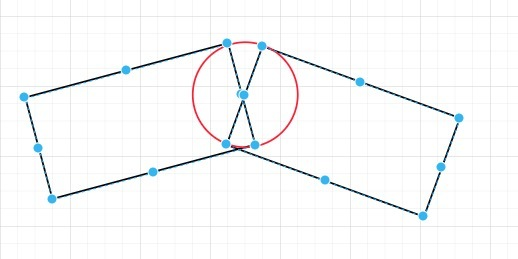https://mp.weixin.qq.com/s/xniwz-a_FI0snWqqPd2NOg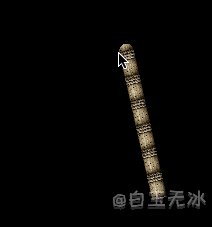## 原创文章导航

2赞

mark 我是卤煮的坚实读者

mark1111111

mark~~~~

mark！！

mark~~~~

mark mark

NewProject.zip (837.8 KB)做了个简单的demo，求大佬们帮忙看看，救救可怜的孩子吧

1赞

1赞